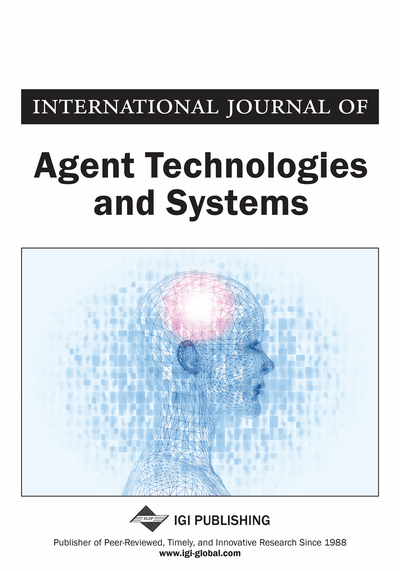# The Meaningfulness of Statistical Significance Tests in the Analysis of Simulation Results

Klaus G. Troitzsch (Universität Koblenz-Landau, Campus Koblenz, Koblenz, Germany)
DOI: 10.4018/IJATS.2016010102

## Abstract

This article discusses the question of whether significance tests on simulation results are meaningful at all. It is also argued that it is the effect size much more than the existence of the effect is what matters. It is the description of the distribution function of the stochastic process incorporated in the simulation model which is important. This is particularly when this distribution is far from normal, which is particularly often the case when the simulation model is nonlinear. To this end, this article uses three different agent-based models to demonstrate that the effects of input parameters on output metrics can often be made “statistically significant” on any desired level by increasing the number of runs, even for negligible effect sizes. The examples are also used to give hints as to how many runs are necessary to estimate effect sizes and how the input parameters determine output metrics.
Article Preview
Top

## Introduction

Multiple runs of simulation models can be seen as samples from an unlimited universe of all possible runs executed by this simulation model for one single parameter combination. In the social sciences, empirical research is usually restricted to only one sample at a time from a limited universe (typically, the population of a country), and this sample is usually biased by effects of the lack of precise information on the universe, low response rates and self-selection. Thus the situation of an empirically active researcher resembles the situation of a researcher running his or her simulation only once for each parameter combination (and usually only for one parameter combination). Only if the empirically active researcher analyses a survey carried out in several countries or regions at the same time with the same questionnaire, his or her counterpart in the simulation community will use several parameter combinations, each corresponding to a different country or region, but even then the empirically active researcher does not know the empirical parameter combination — he or she can give this parameter combination a name, such as, in example 3 below, the name of one of the provinces in Southern Italy. Thus simulation researchers are luckier than their empirically active colleagues, as the latter have to assume some probability distribution for the parameters they want to estimate from their empirical data — and usually they choose a normal distribution or some distribution derived from the normal distribution (such as the χ2 distribution) or use some non-parametric analysis, whereas simulation researchers can construct an arbitrary large “sample of samples” from which they can at least visualise the form of the probability density function of the macro output parameter in question, as this resembles the histogram of the parameter estimates from the individual simulation runs for an identical input parameter combination. And another advantage of simulation researchers is that they have full control of the input parameters of their simulation models whereas even the best controlled lab experiments with human test persons cannot avoid systematic bias (for the complementarity of real-world experiments and “simulation experiments” see also (Beisbart & Norton, 2012)).

If simulation serves the purpose of generating macrostructures from microspecifications (Axtell & Epstein, 1996) (Epstein, 2006, p. 8) then simulation is a deductive attempt (Epstein, 2006, p. xiv): trying to deduce macro behaviour from theoretical assumptions on micro behaviour — which more often than not is not directly observable in real-world scenarios, whereas the macro structure and the macro processes can often be easily observed. Thus, one could argue that a simulation model can be used to test theories on the macro effect of micro assumptions: if the macrostructures predicted by a simulation model do not have empirical correlates then the micro assumptions are at least questionable (for more about the analysis of emergence in multilevel simulation models see also (Castelfranchi, 1998) (Gilbert, et al., 2006)).

The rest of this paper gives several examples of multiple runs of some simulation models and argues that — unlike most empirical analyses — here the question is not whether a certain input parameter (the analogue of an independent variable) — has or has not an effect on a certain output parameter (the analogue of a dependent variable) but what is interesting in simulation research is the form of the relation between input and output parameters and the size of the effect. Sensitivity analysis, of course, is still interested in which input parameters have an effect on which output parameters, but mostly in order to eliminate input parameters whose effect is small.

The examples are taken from a well-published and relatively simple problem: the El Farol Problem (Arthur, 1994), and two much more complex ones one of which extends the famous sugarscape model (Axtell & Epstein, 1996) with an element of networks between agents (König, Möhring, & Troitzsch, 2003) whereas the other is taken from a project enquiring into the social dimensions of organised crime (Troitzsch, et al., 2016) (Troitzsch, 2016). The example models are more complex than the ones analysed for similar purposes by (Currie & Cheng, 2013) or (Goedemé, Van den Bosch, Salanauskaite, & Verbist, 2013) or (Law, 2010) (see also (Hofmann, 2016) whose paper deals with our topic without using examples).

## Complete Article List

Search this Journal:
Reset
Open Access Articles: Forthcoming
Volume 9: 1 Issue (2017)
Volume 8: 1 Issue (2016)
Volume 7: 3 Issues (2015)
Volume 6: 4 Issues (2014)
Volume 5: 4 Issues (2013)
Volume 4: 4 Issues (2012)
Volume 3: 4 Issues (2011)
Volume 2: 4 Issues (2010)
Volume 1: 4 Issues (2009)
View Complete Journal Contents Listing